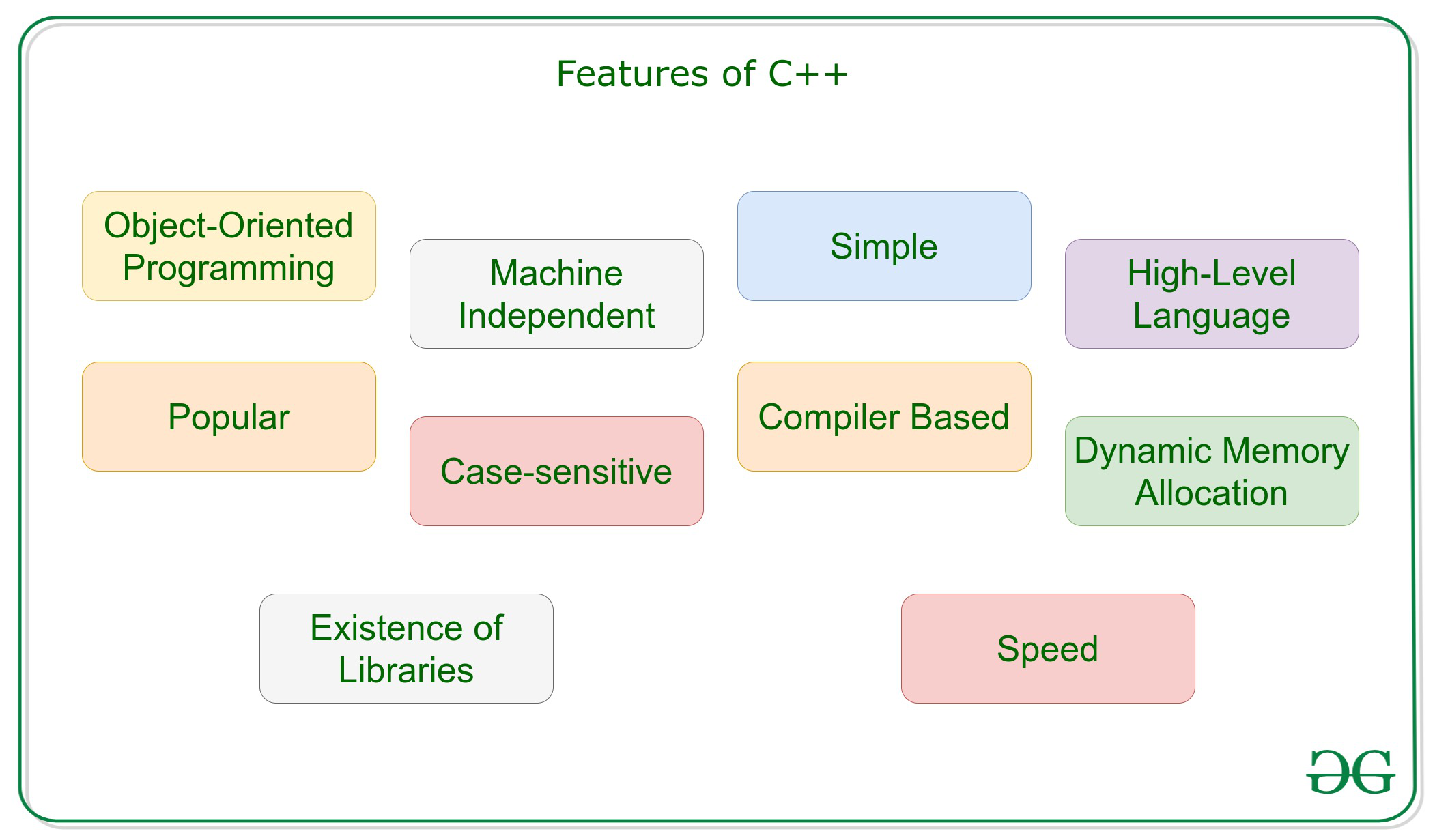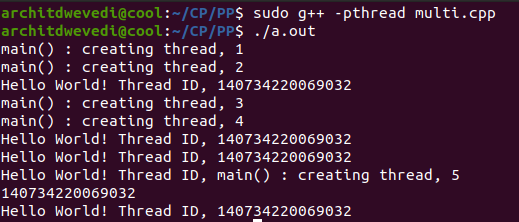# Features of C++

C++ is a general-purpose programming language that was developed as an enhancement of the C language to include object-oriented paradigm. It is an imperative and a compiled language.### Object-Oriented Programming

C++ is an Object-Oriented Programming Language, unlike C which is a procedural programming language. This is the most important feature of C++. It can create/destroy objects while programming. Also, It can create blueprints with which objects can be created. We have discussed the Object-Orient Programming Concepts in C++ in this article.

Concepts of Object-oriented programming Language:

### Machine Independent

A C++ executable is not platform-independent (compiled programs on Linux won’t run on Windows), however they are machine independent.

Let us understand this feature of C++ with the help of an example. Suppose you have written a piece of code which can run on Linux/Windows/Mac OSx which makes C++ Machine Independent but the executable file of the C++ cannot run on different operating systems.

### Simple

It is a simple language in the sense that programs can be broken down into logical units and parts, has rich library support, and a variety of data-types. Also, the Auto Keyword of the C++ makes life easier.

The auto keyword

The idea of the auto was to form the C++ compiler deduce the data type while compiling instead of making you declare the data type every-freaking-time. Do keep in mind that you cannot declare something without an initializer. There must be some way for the compiler to deduce your type.

## C++

 `// C++ program for usin auto keyword ` ` `  `#include ` `using` `namespace` `std; ` ` `  `// Driver Code ` `int` `main() ` `{ ` ` `  `    ``// Variables ` `    ``auto` `an_int = 26; ` `    ``auto` `a_bool = ``false``; ` `    ``auto` `a_float = 26.24; ` `    ``auto` `ptr = &a_float; ` ` `  `    ``// Print typeid ` `    ``cout << ``typeid``(a_bool).name() << ``"\n"``; ` `    ``cout << ``typeid``(an_int).name() << ``"\n"``; ` `    ``return` `0; ` `} `

Output:

```b
i
```

### High-Level Language

C++ is a High-Level Language, unlike C which is a Mid-Level Programming Language. It makes life easier to work in C++ as it is a high-level language as it is closely associated with the human-comprehensible English language.

### Popular

C++ can be the base language for many other programming languages that supports the feature of object-oriented programming. Bjarne Stroustrup found Simula 67, the first object-oriented language ever, lacking simulations and decided to develop C++.

### Case-sensitive

It is clear that the C++ is a case-sensitive programming language. For example, cin is used to take input from the input stream. But if the “Cin” won’t work. Other languages like HTML and MySQL are not case-sensitive language.

### Compiler Based

C++ is a compiler-based language, unlike Python. That is C++ programs used to be compiled and their executable file is used to run it. Due to which C++ is a relatively faster language than Java and Python.

### Dynamic Memory Allocation

When the program executes in the C++ then the variables are allocated the dynamical heap space. Inside of the functions the variables are allocated in the stack space. Many times, We are not aware in advance that how much memory is needed to store particular information in a defined variable and the size of required memory can be determined at run time.

### Memory Management

• C++ allows us to allocate the memory of a variable or an array in run time. This is known as Dynamic Memory Allocation.
• In other programming languages such as Java and Python, the compiler automatically manages the memories allocated to variables. But this is not the case in C++.
• In C++, the memory must be de-allocate the dynamically allocated memory manually after it is of no use.
• The allocation and deallocation of the memory can be done usin the new and delete operators respectively.

Below is the program to illustrate the Memory Management in C++:

## C++

 `// C++ implementation to illustrate ` `// the Memory Management ` ` `  `#include ` `#include ` `using` `namespace` `std; ` ` `  `// Driver Code ` `int` `main() ` `{ ` `    ``int` `num = 5; ` `    ``float``* ptr; ` ` `  `    ``// Memory allocation of ` `    ``// num number of floats ` `    ``ptr = ``new` `float``[num]; ` ` `  `    ``for` `(``int` `i = 0; i < num; ++i) { ` `        ``*(ptr + i) = i; ` `    ``} ` ` `  `    ``cout << ``"Display the GPA of students:"` `         ``<< endl; ` `    ``for` `(``int` `i = 0; i < num; ++i) { ` ` `  `        ``cout << ``"Student"` `<< i + 1 ` `             ``<< ``": "` `<< *(ptr + i) ` `             ``<< endl; ` `    ``} ` ` `  `    ``// Ptr memory is released ` `    ``delete``[] ptr; ` ` `  `    ``return` `0; ` `} `

Output:

```Display the GPA of students:
Student1: 0
Student2: 1
Student3: 2
Student4: 3
Student5: 4
```

• Multithreading is a specialized form of multitasking and multitasking is a feature that allows your system to execute two or more programs concurrently. In general, there are two sorts of multitasking: process-based and thread-based.
• Process-based multitasking handles the concurrent execution of programs. Thread-based multitasking deals with the multiprogramming of pieces of an equivalent program.
• A multithreaded program contains two or more parts that will run concurrently. Each a part of such a program is named a thread, and every thread defines a separate path of execution.
• C++ doesn’t contain any built-in support for multithreaded applications. Instead, it relies entirely upon the OS to supply this feature.

Below is the program to illustrate Multithreading in C++:

## C++

 `// C++ implementation to illustrate ` `// the working of Multi-threading ` ` `  `#include ` `#include ` `#include ` ` `  `using` `namespace` `std; ` ` `  `#define NUM_THREADS 5 ` ` `  `// Function to print Hello with ` `// the thread id ` `void``* PrintHello(``void``* threadid) ` `{ ` `    ``// Thread ID ` `    ``long` `tid; ` `    ``tid = (``long``)threadid; ` ` `  `    ``// Print the thread ID ` `    ``cout << ``"Hello World! Thread ID, "` `         ``<< tid << endl; ` ` `  `    ``pthread_exit(NULL); ` `} ` ` `  `// Driver Code ` `int` `main() ` `{ ` ` `  `    ``// Create thread ` `    ``pthread_t threads[NUM_THREADS]; ` `    ``int` `rc; ` `    ``int` `i; ` ` `  `    ``for` `(i = 0; i < NUM_THREADS; i++) { ` ` `  `        ``cout << ``"main() : creating thread, "` `             ``<< i << endl; ` ` `  `        ``rc = pthread_create(&threads[i], ` `                            ``NULL, ` `                            ``PrintHello, ` `                            ``(``void``*)&i); ` ` `  `        ``// If thread is not created ` `        ``if` `(rc) { ` `            ``cout << ``"Error:unable to"` `                 ``<< ``" create thread, "` `                 ``<< rc << endl; ` ` `  `            ``exit``(-1); ` `        ``} ` `    ``} ` ` `  `    ``pthread_exit(NULL); ` `} `

Output:This tutorial assumes that you are working on Linux OS and we are going to write a multi-threaded C++ program using POSIX. POSIX Threads or Pthreads provides API which is available on many Unix-like POSIX systems such as FreeBSD, NetBSD, GNU/Linux, Mac OS X, and Solaris.

Rated as one of the most sought after skills in the industry, own the basics of coding with our C++ STL Course and master the very concepts by intense problem-solving.

My Personal Notes arrow_drop_upCheck out this Author's contributed articles.

If you like GeeksforGeeks and would like to contribute, you can also write an article using contribute.geeksforgeeks.org or mail your article to contribute@geeksforgeeks.org. See your article appearing on the GeeksforGeeks main page and help other Geeks.

Please Improve this article if you find anything incorrect by clicking on the "Improve Article" button below.

Article Tags :
Practice Tags :

Be the First to upvote.

Please write to us at contribute@geeksforgeeks.org to report any issue with the above content.